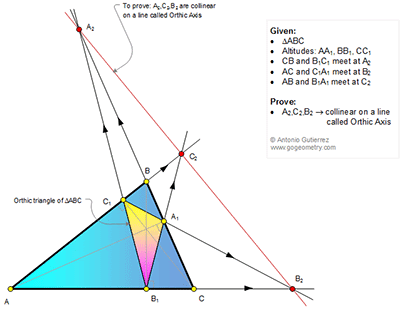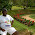## Wednesday, July 6, 2016

### Geometry Problem 1232: Triangle, Altitudes, Orthic Triangle, Collinearity, Orthic Axis

Geometry Problem. Post your solution in the comment box below.
Level: Mathematics Education, High School, Honors Geometry, College.

Click the figure below to view more details of problem 1232.1.https://goo.gl/photos/1o6iZtQfZnuheshYA

Consider complete quadrilateral ABC C1A1B2
Diagonals AA1 and CC1 meet at H
So ( ACB1B2)= -1 or B1, B2 are harmonic conjugate points of A and C…..
Or B2A/B2C=B1A/B1C…. (1)
Similarly we also have A2C/A2B= A1C/A1B…… (2)
And C2B/C2A=C1B/C1A….. (1)
Multiply expressions (1), (2) and (3) side by side
The value of right hand side is 1 per Ceva theorem
So the left hand side B2A/B2C x A2C/A2B x C2B/C2A= 1
And A2, C2, B2 are collinear per inverse of Menelaus theorem

Note: This property can be generalized for any lines AA1, BB1, CC1 as long as that these lines concur at a point

2.Easily A1A2, B1B2 and C1C2 are external angle bisectors of the Orthic Triangle A1B1C1

These angle bisectors meet the opposite sides at A2, B2 and C2

From the result of Problem 631 these points are collinear

Sumith Peiris
Moratuwa
Sri Lanka